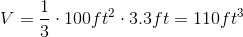## Example Questions

### Example Question #4 : How To Find The Volume Of A Pyramid

What is the sum of the number of vertices, edges, and faces of a square pyramid?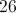Explanation:

A square pyramid has one square base and four triangular sides.

Vertices (where two or more edges come together):  5. There are four vertices on the base (one at each corner of the square) and a fifth at the top of the pyramid.

Edges (where two faces come together):  8. There are four edges on the base (one along each side) and four more along the sides of the triangular faces extending from the corners of the base to the top vertex.

Faces (planar surfaces):  5. The base is one face, and there are four triangular faces that form the top of the pyramid.

Total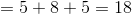### Example Question #5 : How To Find The Volume Of A Pyramid

An architect wants to make a square pyramid and fill it with 12,000 cubic feet of sand. If the base of the pyramid is 30 feet on each side, how tall does he need to make it?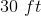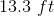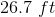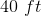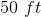Explanation:

Volume of Pyramid = 1/3 * Area of Base * Height

12,000 ft3 = 1/3 * 30ft * 30ft * H

12,000  = 300 * H

H = 12,000  / 300 = 40

H = 40 ft

### Example Question #1 : How To Find The Volume Of A Pyramid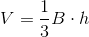, wherestands for base area andfor height.

Find the volume of the pyramid: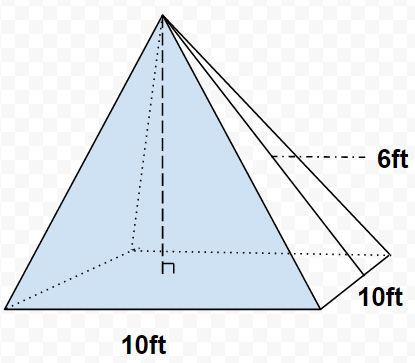The figure is not to scale.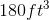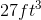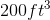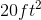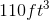Explanation:A helpful strategy for this kind of a problem is to ask the following:
What information am I missing?
What information am I given?
Can I calculate for an unknown variable with the information I have?

The formula given is composed of the Base Area times the height of the pyramid times one-third. This means that in order to find the volume of this pyramid, the area of the square base must be calculated as well as the height of the pyramid (represented by the dashed line in the center). This will allow us to get an answer with cubed feet for units - an inkling that the volume was being calculated and not just the area. So the given equation may be expanded into: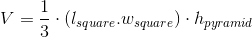Calculating the base area:
Even though pyramids have a square base on which they're commonly seen to be standing on, the base area is calculated for the triangular face. Note that all the triangles are the same.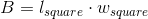will be used to calculate the base area.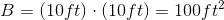Calculating the height of the pyramid:
The height of the pyramid is slightly more tricky than calculating B. Imagine creating a right triangle within the pyramid, which resembles a slpice from the exterior of one of the sides of the pyramid to the center, where the height would be measured. Such triangle is outlined by the dash marks in the following figure: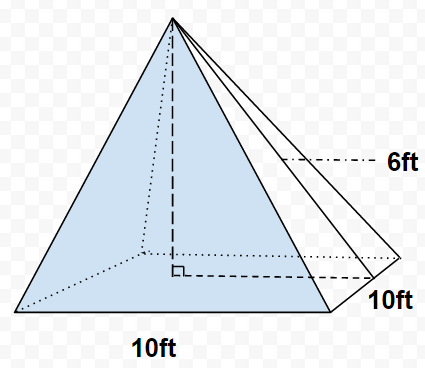The hypotenuse of this triangle would be the height of the triangular face. The pyramid's height becomes to be one of the legs of the triangle - this is our unknown, and may be labeled as x. The other leg of the triangle is 5ft because the leg only extends the length of half of the base of one of the triangles (from one edge to the center).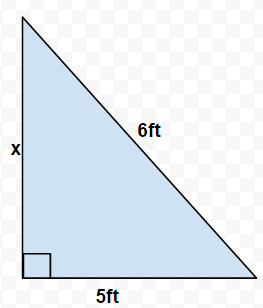Because there are no angles given and two sides of the right triangle can be deduced, x (h of pyramid) can be calculated through the Pythogorean Theorem.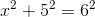, where x=-3.3, +3.3. However, distance cannot be negative so x=-3.3 is not a viable option and the height is left to be as 3.3ft.

Now all information may be substituted into the given equation to solve for the final volume: